Courses

# Test: Motion in a Straight Line- 2

## 20 Questions MCQ Test Physics For JEE | Test: Motion in a Straight Line- 2

Description
This mock test of Test: Motion in a Straight Line- 2 for JEE helps you for every JEE entrance exam. This contains 20 Multiple Choice Questions for JEE Test: Motion in a Straight Line- 2 (mcq) to study with solutions a complete question bank. The solved questions answers in this Test: Motion in a Straight Line- 2 quiz give you a good mix of easy questions and tough questions. JEE students definitely take this Test: Motion in a Straight Line- 2 exercise for a better result in the exam. You can find other Test: Motion in a Straight Line- 2 extra questions, long questions & short questions for JEE on EduRev as well by searching above.
QUESTION: 1

### A particle is projected with a velocity v, so that its range on a horizontal plane is twice the greatest height attained. If g is acceleration due to gravity, then its range is

Solution: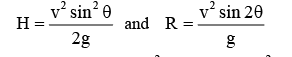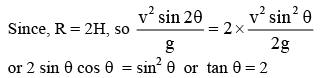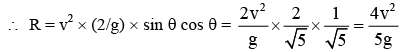QUESTION: 2

### A particle is fired with velocity v making an angle q with the horizontal. What is the magnitude of change in velocity when it is at the highest point?

Solution:

velocity at the highest point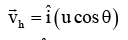velocity at the starting point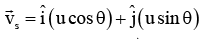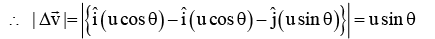QUESTION: 3

### A person can throw a stone to a maximum distance of 100 m. The greatest height to which he can throw the stone is

Solution:

Maximum height is given by H =2gu2sin2θ​

Range is given as R =gu2sin2θ​=100m(given)

Maximum height is attained at 45 degree launch, so θ=45

u2=1000 and Maximum height is 25 m by formula of H.

QUESTION: 4

The ceiling of a hall is 40 m high. For maximum horizontal distance, the angle at which the ball may be thrown with a speed of 56 m/s without hitting the ceiling of the hall is

Solution: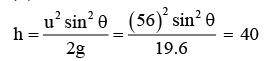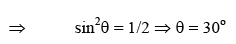QUESTION: 5

A bomb is dropped from an aeroplane when it is directly above a target at a height of 1254.4 m. The aeroplane is going horizontally with a speed of 150 m/s. The distance by which it will miss the target is

Solution:

using the formula:
D = u√2H/g
Where D is distance, u is initial velocity, H is height and g is acceleration due to gravity.
Putting the values,
D= 150√2×1254.4/9.8
D= 150√2508.8/9.8
D= 150√256
D= 150×16
D= 2400m
D= 2.4 km

QUESTION: 6

The  range R of projectile is same when its maximum height are h1 and h2. What is the relation between R, h1 and h2?

Solution:

Range is same for angles of projection θ and 900 - θ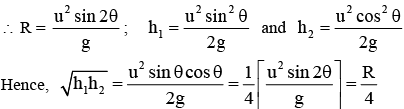QUESTION: 7

A projectile is fired from level ground at an angle θ above the horizontal. The elevation angle of the highest point as seen from the launch point is related to θ by the relation

Solution:

It is clear from the adjoining figure,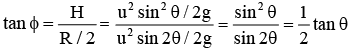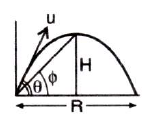QUESTION: 8

A projectile has a time of flight T and range R. For a given angle of projection if the time of flight is doubled, then what happens to the range?

Solution: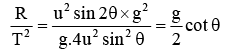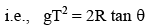If T is doubled, then R becomes 4 times.

QUESTION: 9

hree particles A, B and C are projected from the same point with the same initial speeds making angles 30o, 45o and 60o respectively with the horizontal. Which of the following statements is correct?

Solution:

when a projectile is projected at an angle θ or at angle ( 90o - θ ) with the horizontal, the horizontal range remains the same. The horizontal range is maximum  when the angle of projection is 45o

QUESTION: 10

A particle is projected from the ground with an initial speed of v at an angle q with horizontal. The average velocity of the particle between its point of projection and highest point of trajectory is

Solution:

Average velocity = Displacement/Time

Here,  H = maximum height =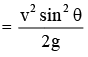R = range =nd T = time of flight =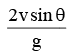QUESTION: 11

With what minimum speed a particle be projected from origin so that it is able to pass through a given point (30 m, 40 m)?

Solution: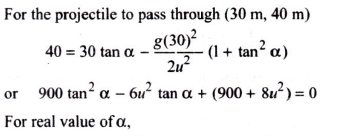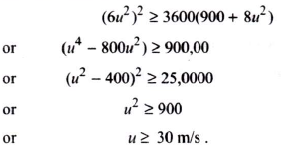QUESTION: 12

A particle is moving along a circular path. The angular velocity, linear velocity, angular acceleration and centripetal acceleration of the particle at any instant respectively are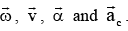. Which of the following relations is not correct?

Solution: Linear velocity is the tangential velocity angular velocity acts in the same direction. So they can never be perpendicular.
QUESTION: 13

A particle is moving along a circular path with uniform speed. Through what angle does its angular velocity change when it completes half of the circular path?

Solution:

Angular velocity is always directed perpendicular to the plane of the circular path. Hence, required change in angle = 0o.

QUESTION: 14

A wheel is subjected to uniform angular acceleration about its axis. Initially its angular velocity is zero. In the first 2 sec, it rotates through an angle q1; in the next 2 sec it rotates through an additional angle θ2, the ratio θ21 is

Solution: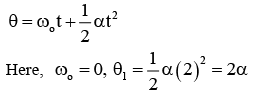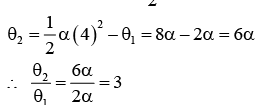QUESTION: 15

If the equation for the displacement of a particle moving on a circular path is given by  θ  = 2t3 + 0.5 where θ is in radian and t in second, then the angular velocity of the particle at  t = 2 s is

Solution: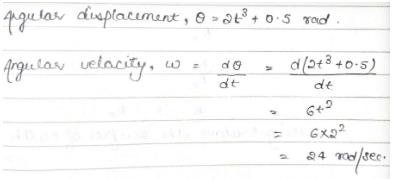QUESTION: 16

Which of the following statements is false for a particle moving in a circle with a constant angular speed?

Solution:

For a particle moving in a circle with constant angular speed, the velocity vector is always tangent to the circle and the acceleration vector always points towards the centre of the circle or is always along the radius of the circle. Since, the tangential vector is perpendicular to radial vector, therefore, velocity vector will be perpendicular to the acceleration vector. But in no case acceleration vector is tangent to the circle.

QUESTION: 17

Which motion does not require force to maintain it?

Solution:

Uniform straight line motion is a type of linear motion. It works according to Newton's First law of motion i.e. objects that do not experience any net force will continue to move in a straight line with a constant velocity until they are subjected to a net force.

QUESTION: 18

Two trains are each 50 m long moving parallel to each other at speeds 10 m/s and 15 m/s respectively, at what time will they pass each other?

Solution:

As both the trains are moving in the opposite direction, the relative speed of each train with respect to each other be, v=10+15=25m/s
Here distance covered by each train = sum of their lengths =50+50=100m
∴ Required time=100/25​=4sec

QUESTION: 19

Two cars are moving in same direction with speed of 30 kmph. They are separated by a distance of 5 km. What is the speed of a car moving in opposite direction if it meets the two cars at an interval of 4 min?

Solution:

Lets say the speed of second car be v. Now with respect to car 1, speed of car 2 is v - 30. Now if the cross each other at just after 4 min then the distance traveled by car 2 relative to car 1 is (v -30) x 4/60 and we know the distance travelled relatively is 5km
Thus we get
(v -30) x 4/60 = 5
V = 300/4 - 30
= 45 km/h

QUESTION: 20

A man can swim in still water at a speed of 6 kmph and he has to cross the river and reach just opposite point on the other bank. If the river is flowing at a speed of 3 kmph, and the width of the river is 2 km, the time taken to cross the river is (in hours)

Solution:

To reach the exact opposite end the parallel component of velocity would nullify the velocity of the river, hence we get 6.sin a = 3 , where a is the angle with perpendicular to river flow.
Thus we get sin a = ½ and cos a =√3/2
Thus the perpendicular component of velocity, 6.cos a =  3√3
Thus time taken is 2 / 3√3